Direct product

(diff) ← Older revision | Latest revision (diff) | Newer revision → (diff)

A basic general mathematical construction. The idea behind it is due to R. Descartes; therefore the direct product is also called the Cartesian product. The direct product, or simply the product, of two non-empty setsandis the set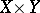consisting of all ordered pairs of the form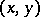,,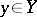: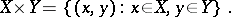If one of the setsoris empty then so is their product. The setcan be identified with the set of functions defined on the two-element set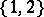and taking the value 1 for elements of, and the value 2 for elements of. This identification leads to a general definition of a direct product of sets. Letbe some index set and suppose that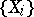is an arbitrary family of sets, indexed by the elements of. The direct product of the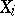,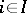, is the set of functions, where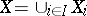, such that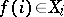for every. Usually, the direct product is denoted by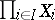, for a finite index set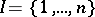one also uses the notations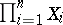and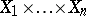. Ifconsists of the single element 1, then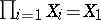. Sometimes one defines the direct product of a finite number of factors inductively: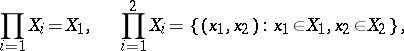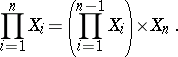One merit of the construction of a direct product rests above all in the possibility of naturally introducing supplementary structures in it, if all factors have the same mathematical structure. E.g., if the,, are algebraic systems of the same type, i.e. sets with a common signature of finitely-placed predicates and operations, then the product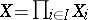can be made into an algebraic system of the same signature: For functions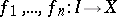and an-ary operationthe action of the functionon one elementis defined by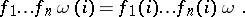The value of a predicate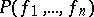is true if for everythe value of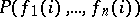is true. Moreover, if in allan equation is satisfied, then it is also satisfied in their product. Therefore, the product of semi-groups, groups, rings, vector spaces, etc., is again a semi-group, group, ring, vector space, respectively.

For an arbitrary factor of a direct productthere exists a natural projection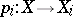, defined by. The setand the family of projections,, have the following universal property: For every family of mappings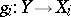there exists a unique mapping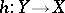such thatfor every. This property also holds if allare algebraic systems of one type, and makes it possible to define a suitable topology on a direct product of topological spaces. The property formulated is the basis for the definition of the product of a family of objects in a category.

One often encounters problems of describing mathematical objects that cannot be decomposed into a direct product, and of stating conditions under which the factors of a direct product are uniquely determined up to an isomorphism. Classical results in this respect are the theorem on the structure of finitely-generated modules over principal ideal rings and the Remak–Schmidt theorem on the central isomorphism of direct decompositions of a group with a principal series.

The direct product is sometimes called the complete direct product, to distinguish it from the discrete direct product (or direct sum), which is defined when a supplementary structure in the factors makes it possible to distinguish one-element substructures (e.g. unit subgroups, null subspaces, etc.). As a rule, the direct product of a finite number of factors coincides with the discrete product.

How to Cite This Entry:
Direct product. Encyclopedia of Mathematics. URL: http://encyclopediaofmath.org/index.php?title=Direct_product&oldid=13729
This article was adapted from an original article by M.Sh. Tsalenko (originator), which appeared in Encyclopedia of Mathematics - ISBN 1402006098. See original article